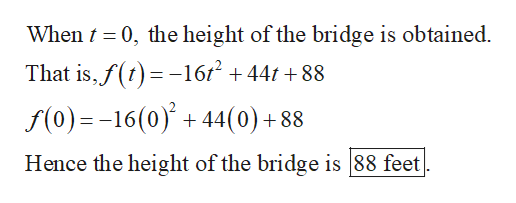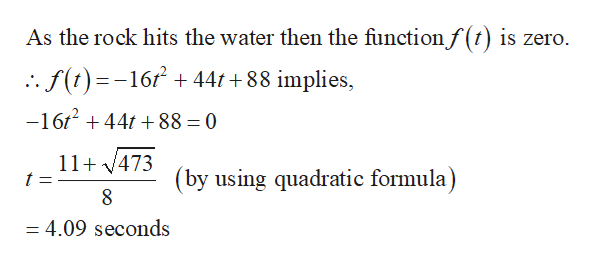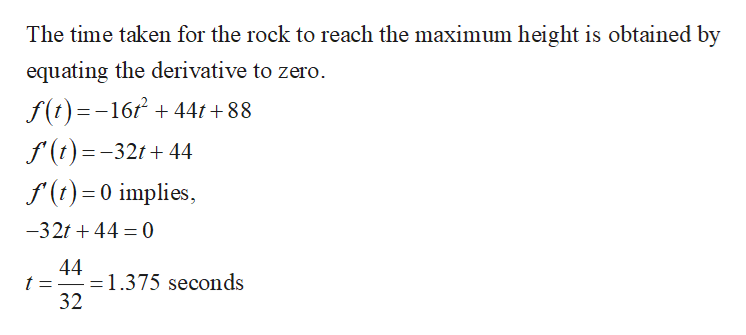# A rock is thrown upward from a bridge into a river below. The function f(t)=−16t2+44t+88 determines the height of the rock above the surface of the water (in feet) in terms of the number of seconds t since the rock was thrown.What is the bridge's height above the water?feet How many seconds after being thrown does the rock hit the water?secondsHow many seconds after being thrown does the rock reach its maximum height above the water?secondsWhat is the rock's maximum height above the water?

Question

A rock is thrown upward from a bridge into a river below. The function f(t)=−16t2+44t+88 determines the height of the rock above the surface of the water (in feet) in terms of the number of seconds t since the rock was thrown.

1. What is the bridge's height above the water?

feet

• How many seconds after being thrown does the rock hit the water?

seconds

• How many seconds after being thrown does the rock reach its maximum height above the water?

seconds

• What is the rock's maximum height above the water?

check_circleExpert Solution
Step 1

It is given that the function f(t) = -16t2+44t+88 determines the height of the rock above the surface of water.

1.The height of the bridge above water is determined.help_outlineImage TranscriptioncloseWhen 0, the height of the bridge is obtained. That is,f( 16/2 +441 +88 f(0)=-16(0)+44(0) + 88 Hence the height of the bridge is 88 feet fullscreen
Step 2

2.The time taken for the rock thrown to hit the water is computed.help_outlineImage TranscriptioncloseAs the rock hits the water then the functionf(t) is zero. .f()-16441 +88 implies, -16t244t88 = 0 11 473 t (by using quadratic formula) 8 = 4.09 seconds fullscreen
Step 3
1. The time taken for the rock to reach the maximum heigh...help_outlineImage TranscriptioncloseThe time taken for the rock to reach the maximum height is obtained by equating the derivative to zero f(t)=-16/244t+88 f(t)=-321+44 f(t)0 implies, -32144 0 44 1.375 seconds 32 fullscreen

### Want to see the full answer?

See Solution

#### Want to see this answer and more?

Solutions are written by subject experts who are available 24/7. Questions are typically answered within 1 hour*

See Solution
*Response times may vary by subject and question
Tagged in

### Other